Working with Angles MathBitsNotebook.com Terms of Use   Contact Person: Donna RobertsIf D lies in the interior of ∠ABC, then m∠ABD + m∠DBC = m∠ABC.

This concept may also be referred to as
"whole quantity" or "the whole is equal to the sum of its parts."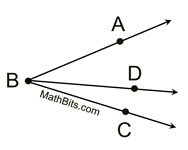Angle Bisector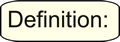An angle bisector is a ray from the vertex of the angle into the interior of the angle forming two congruent angles (angles of equal measure).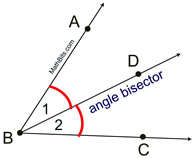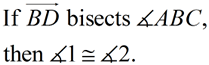Remember that the word "bisect" means to cut into two equal pieces.

 Angles Forming a Straight Line

 If the non-shared sides of two, or more, adjacent angles form a straight line, the measures of the angles add up to 180º.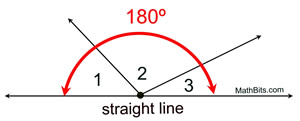m∠1 + m∠2 + m∠3 = 180º This is a "common sense" rule. A straight line is also a straight angle, which contains 180º. If these angles are adjacent (don't overlap), and their non-shared sides form a straight line, the angles comprise a straight angle which contains 180º.

 Angles Around a Point

 If two, or more, adjacent angles completely surround a point, the measures of the angles add up to 360º.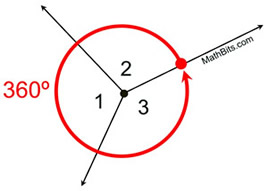m∠1 + m∠2 + m∠3 = 360º This is also a "common sense" rule. The complete revolution around a point is a circular 360º. If these angles are adjacent (don't overlap), and together they form one complete revolution, the measures of the angles will total 360º.